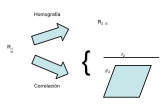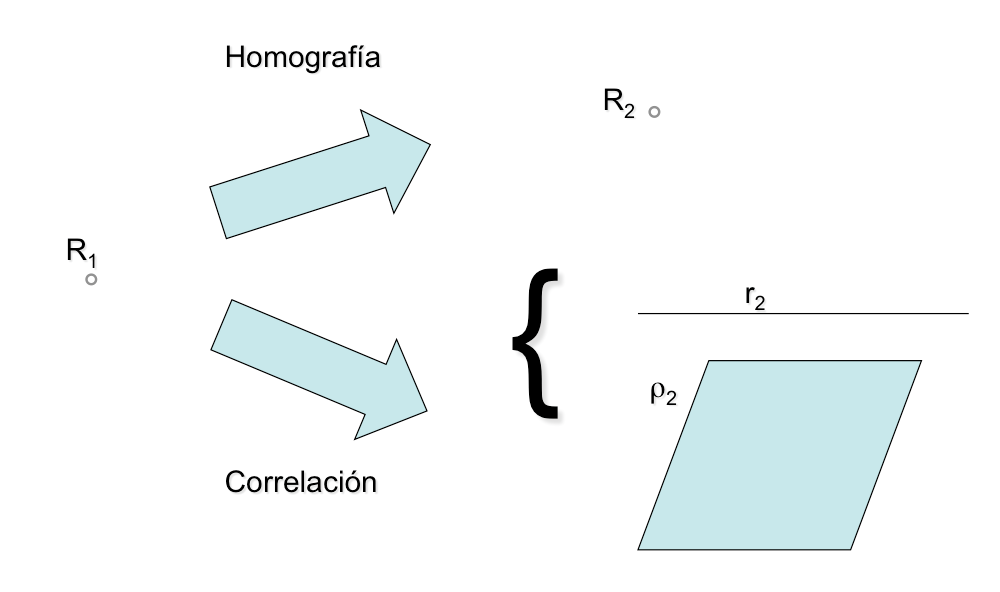# Geometric transformations : Correlations Vs homographiesGeometric transformations can be understood as a set of geometric operations that create a new figure from a previously given, invariants and properties obtained in these. The new figure is called “homologous” or correlative of the original depending on the nature of the transformation of its basic elements.

A homography is a transformation that preserves the nature of the elements processed.
• One point is transformed into another point
• A straight line is transformed into another
• A plane is transformed into another plane
A correlation is a transformation that NO preserves the nature of the elements processed.
• A point can be transformed into a straight or flat, but not elsewhere
• A line can be transformed into a point or plane, but not in another line
• A plane can be transformed into a line or point, but on another levelIn the figure above these concepts are outlined. A certain element, for example a point, transforms into another element of the same nature, point, using a homography, while if we make the transformed correlation may be a straight or flat but never a point.
Methodology of a geometric transformation
When we study a geometric transformation must systematically analyze a series of sections that will give us sufficient knowledge of the same.
• Transform definition
• Transformation of basic elements
• Do you keep the form? (Such is)
• Do you keep the angles (As is )?
• ¿Es involutiva?
• Properties
• Main applications
The definition of the transformation must include the analysis of the number of parameters or restrictions necessary for proper determination, So, a translation must be defined by an address and a value to indicate module or the distance between two homologous points or processed, but also will be defined by determining a point and the transformed. We see that the same transformation can thus be determined with different data.
We will discuss how to get processed for each of the basic elements: points, straight, circunferencias …. as a geometric shape can be resolved into these elements to be transformed.
The invariant or projective metric aspects and that remain in the transformation will be used to simplify the necessary operations used in the transformation, and to determine their potential applications in solving geometric problems.
In particular is of special interest to know the behavior of the angular relationships; if a line and are parallel transformed and if the angle between two elements is maintained in the transformation (conformal transformations).
In particular in the case of homographies is interesting to determine whether the transformation is involutive, namely, whether to apply the transformation to become an element of the original element is obtained. For example, a translation is not involutive, since applying the movement from one point to become a different point is obtained, while if it is involutive symmetry. (Not to be confused with the inverse involution transformation).